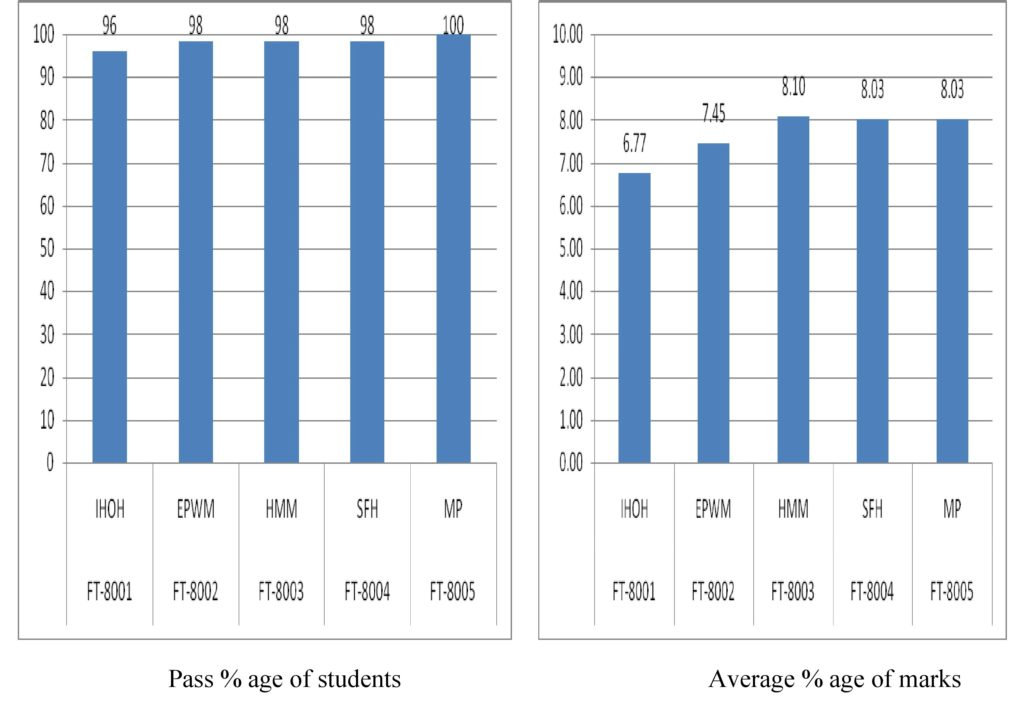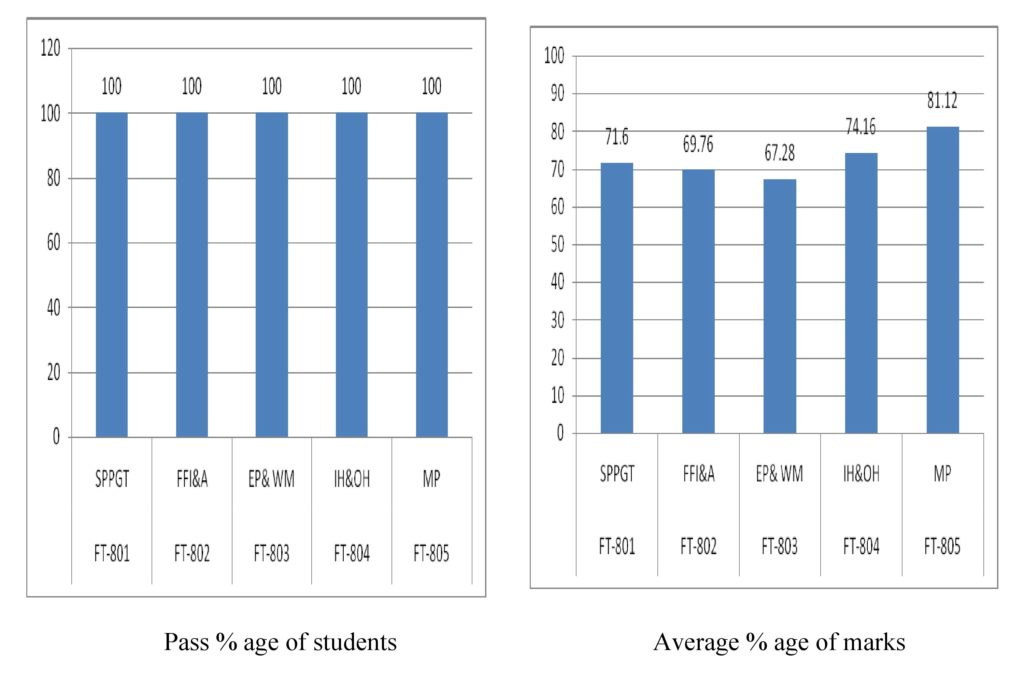Result Analysis

FIRE TECHNOLOGY & SAFETY ENGINEERING

Final Year 2015- 2019 Batch

 Top five students Sr. No. Student Name = CGPA 1 Arpita Roy = 8.36 2 Rahul Singh = 8.13 3 Matte Praveen Kumar = 8.11 4 Gaurav Bhawsar = 8.10 5 Sujith Kumar = 8.03 Pass percentage = 94.40% Total Students Appearing = 125 No. of students pass = 118 No. of student passed with Hons. = 27 No. of students passed in I Div. = 70 No. of students passed in II Div. = 21Final Year 2014- 2018 Batch
 Top five students Sr. No. Student Name = CGPA 1 Rishabh Mishra = 8.41 2 Mahendra Singh Shaktawat = 8.39 3 Aman Porwal = 8.10 4 Bhagya Shree Sharma = 8.06 5 Jay Singh Manral = 8.06 Pass percentage = 100% Total Students Appearing = 125 No. of students pass = 103 No. of student passed with Hons. = 29 No. of students passed in I Div. = 48 No. of students passed in II Div. = 26